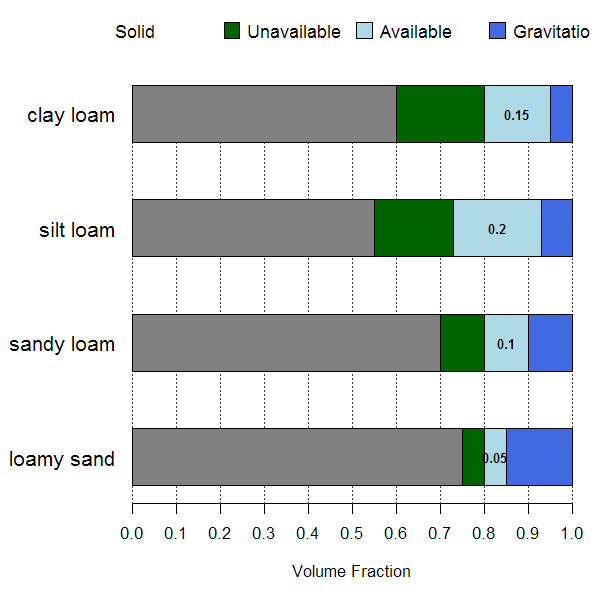plotAvailWater {sharpshootR} R Documentation

## Visual Demontration of Available Soil Water

### Description

Generate a simplistic diagram of the various fractions of water held within soil pore-space.

### Usage

```plotAvailWater(x, width = 0.25, cols = c(grey(0.5),
"DarkGreen", "LightBlue", "RoyalBlue"), name.cex = 0.8)
```

### Arguments

 `x` a `data.frame` containing sample names and water retention data, see examples below `width` vertical width of each bar graph `cols` a vector of colors used to symbolize 'solid phase', 'unavailable water', 'available water', and 'gravitational water' `name.cex` character scaling of horizon names, printed on left-hand side of figure

D.E. Beaudette

### Examples

``````# demonstration
s <- data.frame(
name=c('loamy sand', 'sandy loam', 'silt loam', 'clay loam'),
pwp=c(0.05, 0.1, 0.18, 0.2),
fc=c(0.1, 0.2, 0.38, 0.35),
sat=c(0.25, 0.3, 0.45, 0.4))
s\$solid <- with(s, 1-sat)

par(mar=c(5, 6, 0.5, 0.5))
plotAvailWater(s, name.cex=1.25)
````````````# use some real data from SSURGO
## Not run:
##D library(soilDB)
##D
##D q <- "SELECT hzdept_r as hztop, hzdepb_r as hzbottom,
##D hzname as name, wsatiated_r/100.0 as sat,
##D wthirdbar_r/100.0 as fc, wfifteenbar_r/100.0 as pwp, awc_r as awc
##D FROM chorizon
##D WHERE cokey IN (SELECT cokey from component where compname = 'dunstone')
##D AND wsatiated_r IS NOT NULL
##D ORDER BY cokey, hzdept_r ASC;"
##D
##D x <- SDA_query(q)
##D x <- unique(x)
##D x <- x[order(x\$name), ]
##D x\$solid <- with(x, 1-sat)
##D
##D par(mar=c(5, 5, 0.5, 0.5))
##D plotAvailWater(x)
## End(Not run)
``````

[Package sharpshootR version 0.8-4 Index]